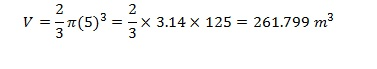# How to Find the Volume of a Sphere

Sphere, approximately, is the shape of an ordinary tennis ball or football. The shape is so common in nature, from the shape of the planets and stars to small water drops. It has significance in engineering and sciences also. Therefore, it is important to know the attributes of spheres and way to measure them. Volume is one such attribute.

Mathematically, the sphere is defined as the surface created by the set of points lying at a constant distance from a fixed point in space, where the constant pit is known as the center, and the distance from the center to the surface is known as the radius. Any object displaying the above mentioned characteristic is said to have a spherical shape. If the inside of the sphere is empty, it is referred to as a spherical shell or a hollow sphere. If the inside of the sphere is filled, it is called as a solid sphere .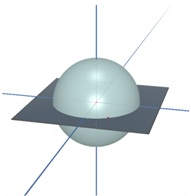## Volume of a Sphere – Formula

The volume of a sphere is given by the formula,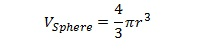This formula was first derived by Archimedes using the result that a sphere occupies 2/3 of the volume of a circumscribed cylinder. A semi- sphere is one half of a complete sphere and volume of a semi-sphere is half of the sphere. Therefore, the volume of the semi-sphere is given by the formula,

## Volume of a Semi-Sphere – Formula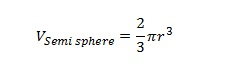These formulas are obtained by integration methods. Consider a sphere with radius of r centered at the origin of the coordinate axes as shown above. A small incremental distance in the x direction is given by dx. A slab of thickness dx will approximately have a cylindrical shape with a radius y. The volume of the cylinder can be given as(dV)=πy^2 dx. Therefore, the volume of the sphere is given by the integral within the limits of the radius,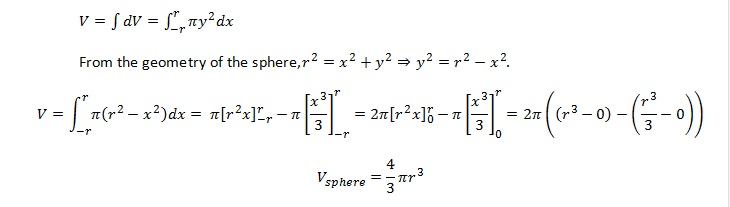To find the volume of the sphere only one measure of the sphere has to be known, which is the radius of the sphere. If diameter is known, the radius can be easily computed using the relation D=2r. After determining the radius, use the formula derived above.

## How to find the volume of a sphere: Example

• The radius of a sphere is 10cm. What is the volume of the sphere?

The radius is given. Therefore, the volume of the sphere can be calculated as follows,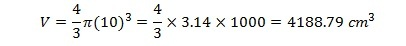## How to find the volume of a semi-sphere: Example

• A spherical shape water tank has a diameter of 5m. If the water is filled at a rate of 5ls-1. If the tank was half filled in the beginning, how long is it going to take to fill the tank completely?

The problem has to be solved in two simple steps. First we have to find the empty volume in the beginning, and then find the time it takes to fill that volume. The tank is half filled initially. Therefore, we have to calculate the volume of a semi-sphere, which is also the volume filled with water.